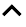or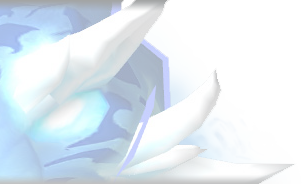Shadowlands Alpha ~~ 5/12/20Subscribe via RSS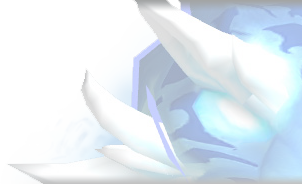2020-05-15 08:26:41
PET NEWS - BY DRAGONSAFTERDARK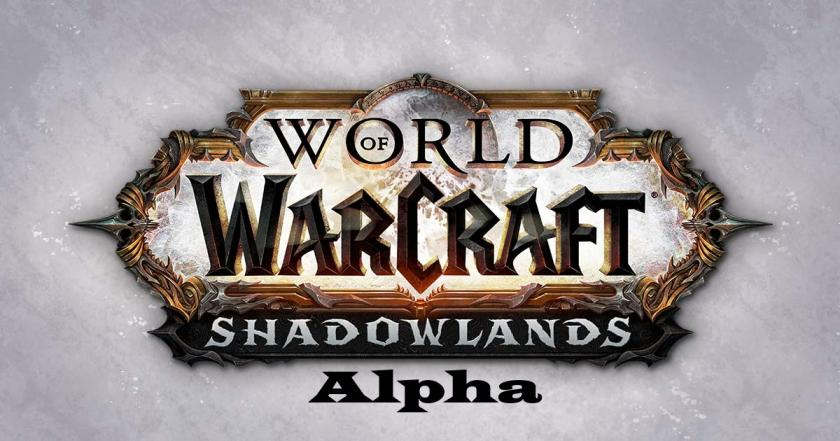Not much has changed or been added to the Shadowlands Alpha as far as pets are concerned, but we do have a few updates.

Before we get into those, though, we have our obligatory spoiler warning:~~~~~

~~~~

~~~

~~

~

And with that out of the way, off we go!

Previous articles:

Datamined Potential Pet Models

• Player Pet Changes
• NPC Pet Changes
• New Abilities -- NPC, Maybe Player Pets Eventually
• Numbers for Stampede-esque & Black Claw Changes
• New Pets / NPC

# 1. Player Pet Changes

Some are changes we've already covered, but a couple are new:

**NOTE: All pets listed below are for RARE quality pets @ 25 **

• Cinder Kitten

1400 HP 292 P 292 S -> 1400 HP 284 P 284 S

• Tiny Blue Carp

1465 HP 289 P 289 S -> 1465 HP 281 P 281 S

• Hermit Crab

1400 HP 284 P 349 S -> 1278 HP 272 P 337 S

• Mechanical Cockroach

1587 HP 260 P 293 S -> 1587 HP 248 P 293 S

• Mechanical Prairie Dog

1587 HP 297 P 260 S -> 1506 HP 297 P 260 S

• Crypt Fiend

1197 HP 349 P 293 S -> 1197 HP 349 P 276 S

• Slimy Darkhunter

massive Speed reduce for all breeds

• Boghopper

1400 HP 284 P 349 S -> 1339 HP 248 P 349 S

# 2. NPC Pet Changes

• Frill (BRD PBD Alran Heartshade)

1438 HP 215 P 301 S -> 1234 HP 189 P 301 S

• Ruddy (BRD PBD Alran Heartshade)

1505 HP 195 P 252 S -> 1285 HP 176 P 252 S

• Wanderer (BRD PBD Alran Heartshade)

1607 HP 221 P 297 S -> 1319 HP 176 P 297 S

• Crushface (BRD PBD Zuna Skullcrush)

1505 HP 221 P 244 S -> 1285 HP 189 P 244 S

• Fozling (BRD PBD Zuna Skullcrush)

1607 HP 234 P 236 S -> 1336 HP 221 P 236 S

• Tremors (BRD PBD Zuna Skullcrush)

1471 HP 234 P 195 S -> 1285 HP 211 P 195 S

• Logic (BRD PBD Therin Skysong)

1505 HP 254 P 297 S -> 1505 HP 221 P 297 S

• Math (BRD PBD Therin Skysong)

1471 HP 221 P 305 S -> 1471 HP 176 P 305 S

# 3. New Abilities

These abilities are attached to what we believe to be a new NPC team pet: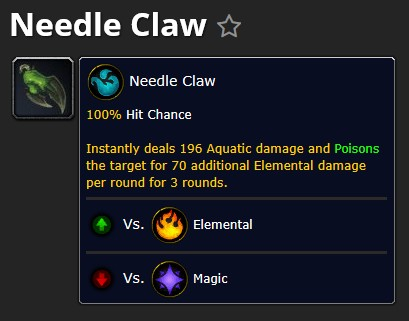This ability is not currently attached to an NPC pet, or a player pet: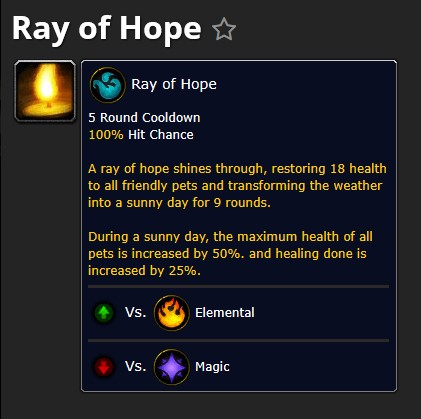# 4. Flock & Black Claw Numbers

The below numbers were provided by Lazey:

Examples (don’t blame me for Blizzard math and rounding weirdness *g*):

• Ikkys’ Flock base damage in the journal is 153 so one of the three hits does 51 damage, ⅔ of that is 34. I will use multipliers 2.0/1.5 for Shattered Defenses pre/post nerf.

Round 1: 3x 51 damage (153 total)
Round 2: 3x 128 damage (51+34 = 85*1.5 = 128 = 384 total)
Round 3: 3x 179 damage (51+34+34 = 119*1.5 = 179 = 539 total)

A pure Flock attack does 1076 in total in Shadowlands.

• On live servers you see this:

Round 1: 3x 51 damage (153 total)
Round 2: 3x 102 damage (51*2,0 = 102 = 308 total)
Round 3: 3x 102 damage (51*2.0 = 102 = 308 total)

769 total damage (without racial multipliers, crits, flat damage increase etc.)

So far it looks like we’ll see a damage increase of Swarm abilities when not using other damage modifiers.

• Now let’s take these examples and add Ikkys’ yet unchanged Black Claw flat 136 damage increase:

Round 1: 3x 188 damage (51+136 = 188x3 = 564 total)
Round 2: 3x 265 damage (51+34 = 85*1.5 = 128+136 = 265x3 = 795 total)
Round 3: 3x 316 damage (51+34+34 = 119*1.5 = 179+136 = 316x3 = 948 total)

So we have 2307 damage in total in Shadowlands.

• On live servers we see:

Round 1: 3x 188 damage (51+136 = 188x3 = 564 total)
Round 2: 3x 376 damage (51+136 = 188*2.0 = 376x3 = 1128 total)
Round 3: 3x 376 damage (51+136 = 188*2.0 = 376x3 = 1128 total)

2820 total damage (without racial multipliers, crits, etc.)

• Ikkys’ Shadowlands Flock with live server Black Claw calculation would be 2709 total damage (probably we will never experience that):

Round 1: 3x 188 damage (51+136 = 188x3 = 564 total)
Round 2: 3x 332 damage (51+34+136 = 221*1.5 = 332x3 = 996 total)
Round 3: 3x 316 damage (51+34+34 = 255*1.5 = 383x3 = 1149 total)

• Now looking into Ikkys' Black Claw + Flock vs. a fictional shield that reduces 119 damage from every attack (one time assuming it being active all the time, second time assuming it’s gone in round 3 of Flock)

Round 1: 3x 69 damage (51-119+136 = 69x3 = 207 total)
Round 2: 3x 146 damage (51+34 = 85*1.5 = 128-119+136 = 146x3 = 438 total)
Round 3: 3x 197 damage (51+34+34 = 119*1.5 = 179-119+136 = 197x3 = 591 total)
Round 3: 3x 315 damage (51+34+34 = 119*1.5 = 179+136 = 315x3 = 945 total)

1236 / 1590 total damage

Live servers:

Round 1: 3x 69 damage (51-119+136 = 69x3 = 207 total)
Round 2: 3x 138 damage (51-119+136 = 69*2.0 = 138x3 = 414 total)
Round 3: 3x 138 damage (51-119+136 = 69*2.0 = 138x3 = 414 total)
Round 3: 3x 376 damage (51+136 = 188*2.0 = 376x3 = 1128 total)

1034 / 1749 total damage

• Checking Ikkys' Black Claw + Flock vs. a stronger fictional shield that reduces 226 damage from every attack (one time assuming it being active all the time, second time assuming it’s gone in round 3 of Flock)

Round 1: 3x blocked (51-226+136 = 0x3 = 0 total, no SD applied)
Round 2: 3x blocked (51+34-226+136 = 0x3 = 0 total, no SD applied)
Round 3: 3x 29 damage (51+34+34-226+136 = 29x3 = 87 total)
Round 3: 3x 255 damage (51+34+34+136 = 255x3 = 765 total)

87 / 852 total damage

Live servers:

Round 1: 3x blocked damage (51-226+136 = 0x3 = 0 total, no SD applied)
Round 2: 3x blocked damage (51-226+136 = 0x3 = 0 total, no SD applied)
Round 3: 3x blocked damage (51-226+136 = 0x3 = 0 total, no SD applied)
Round 3: 3x 188 damage (51+136 = 188x3 = 564 total)

0 / 564 total damage

• And another Ikkys' Black Claw + Flock vs. a weaker fictional shield that reduces 66 damage from every attack (one time assuming it being active all the time, second time assuming it’s gone in round 3 of Flock)

Round 1: 3x 121 damage (51-66+136 = 121x3 = 363 total)
Round 2: 3x 198 damage (51+34 = 85*1.5 = 128-66+136 = 198x3 = 594 total)
Round 3: 3x 249 damage (51+34+34 = 119*1.5 = 179-66+136 = 249x3 = 747 total)
Round 3: 3x 315 damage (51+34+34 = 119*1.5 = 179+136 = 315x3 = 945 total)

1704 / 1902 total damage

Live servers:

Round 1: 3x 121 damage (51-66+136 = 121x3 = 363 total)
Round 2: 3x 138 damage (51-66+136 = 121*2.0 = 242x3 = 726 total)
Round 3: 3x 138 damage (51-66+136 = 121*2.0 = 242x3 = 726 total)
Round 3: 3x 376 damage (51+136 = 188*2.0 = 376x3 = 1128 total)

1815 / 2217 total damage

# 5. New Pets

## 5.1 Potential New Player Pets

So, the Pocket Colossus was replaced by the Pocket PHA7-YNX on the Alpha. However, their models are incredibly different, and they have different flavor text, so we might see the Colossus come back.

Pocket Colossus replaced by:
Pet Species ID: 2889
Profession: Engineering
“Inspired by the Kyrian Phalynx, Engineers have modified the design to be approximately 300% more adorable.”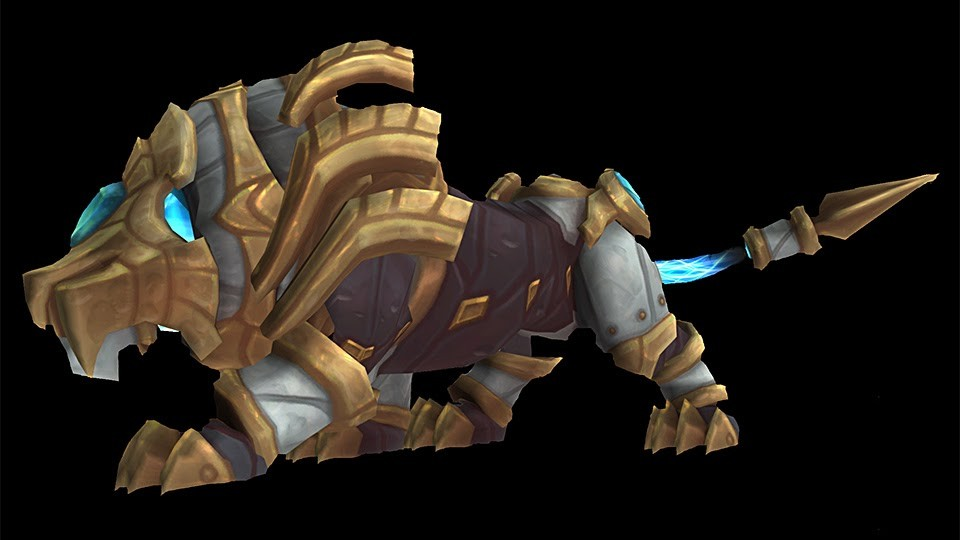Pocket Colossus
Pet Species ID: 2889
Profession: Engineering
“Inspired by the Kyrian Colossus, Engineers have modified the design to be much more diminuitive.”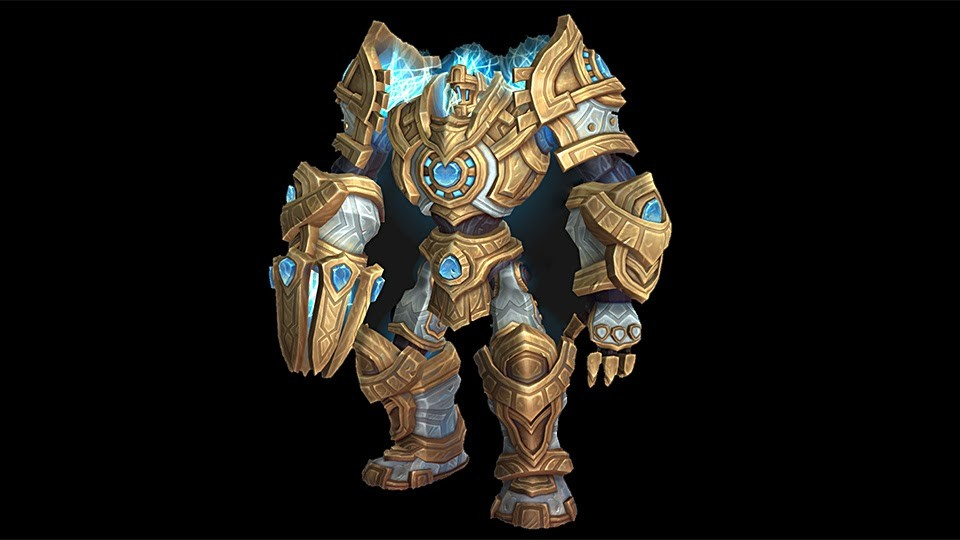## 5.2 Potential New NPC & Team

Glimr -- Might be the tamer / enemy NPC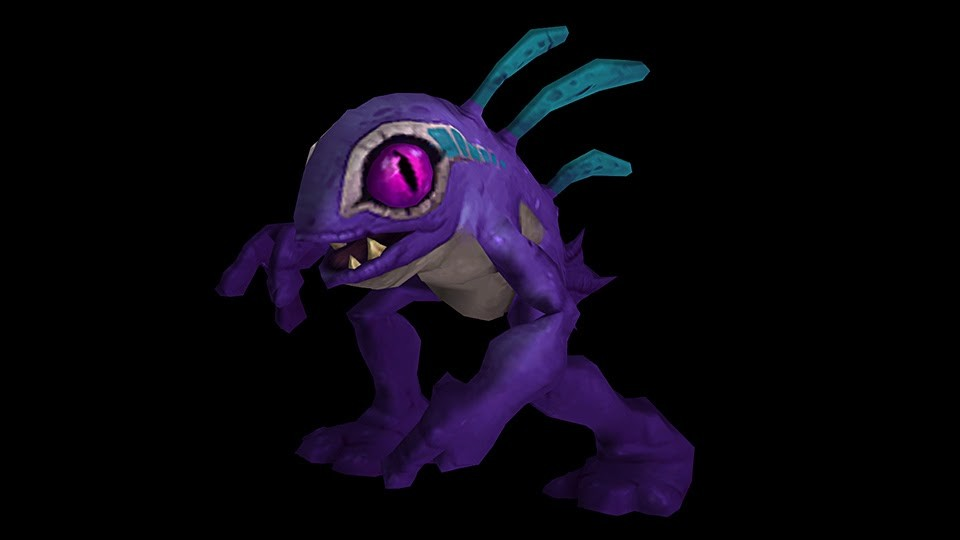Potential Team:

Whipper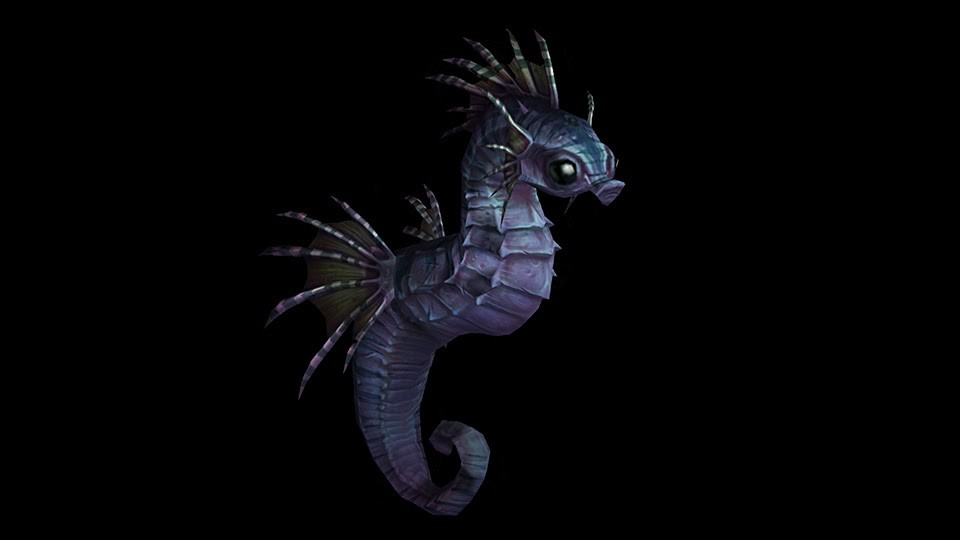Snipper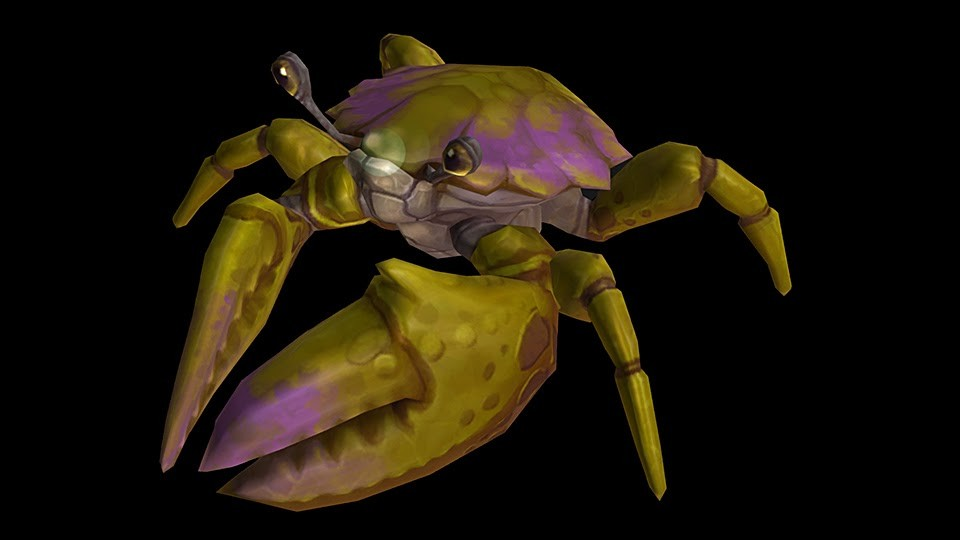Flipper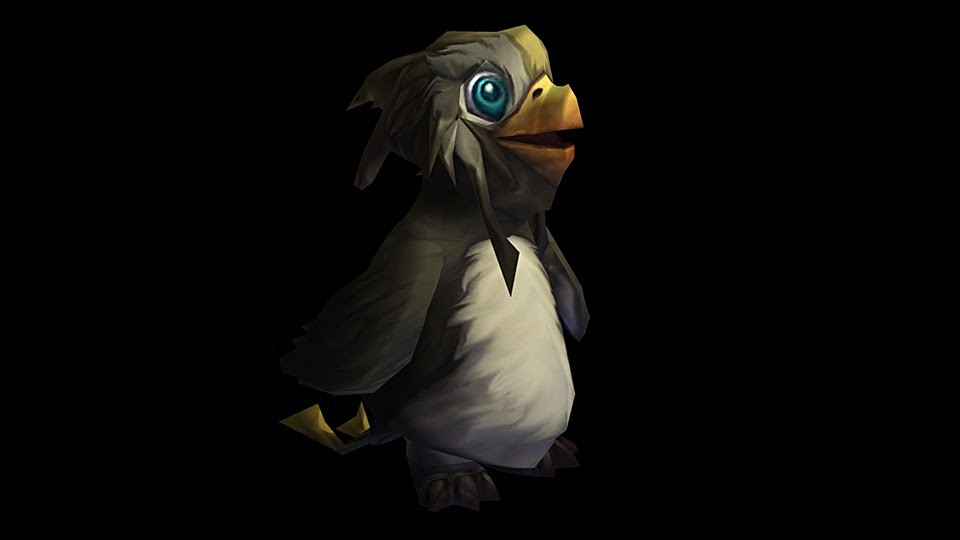That's all we have for now!

How do you feel about some of the preliminary Flock / Black Claw numbers? Do you still feel confident that pets that Ikky will be able to pull their weight on the tougher battles? Let us know below!

We hope you're all doing well, and until next time: happy battling!

 3 Comment (EN) Show first: Newest Oldest Highest rating Lowest rating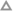You must be logged in to vote on comments 1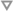You must be logged in to vote on comments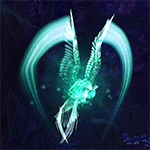wrote on 05/17/2020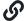As for the Ikky nerf, we knew it was coming. I think we probably will have to be utilizing more DoT damages that run in sync with Shattered Defenses (Turrets, Make It Rain, etc) to offset the overall Flock+SH damage nerf.
The last 4 Battle Pets you have listed, I wonder if maybe those might be 2021 Shadowlands Children's Week rewards?You must be logged in to vote on comments 1You must be logged in to vote on commentswrote on 05/17/2020Snipper has abilities seen only on enemy NPC pets thus far, so unless they're also adding new abilities to the player pet pool, chances are they're an NPC team. :)You must be logged in to vote on comments 1You must be logged in to vote on commentswrote on 05/18/2020You are correct. I honestly did not look at the abilities and since it's a set of 3 abilities and not 6, yeah. (edited)